• Stream
• Portfolio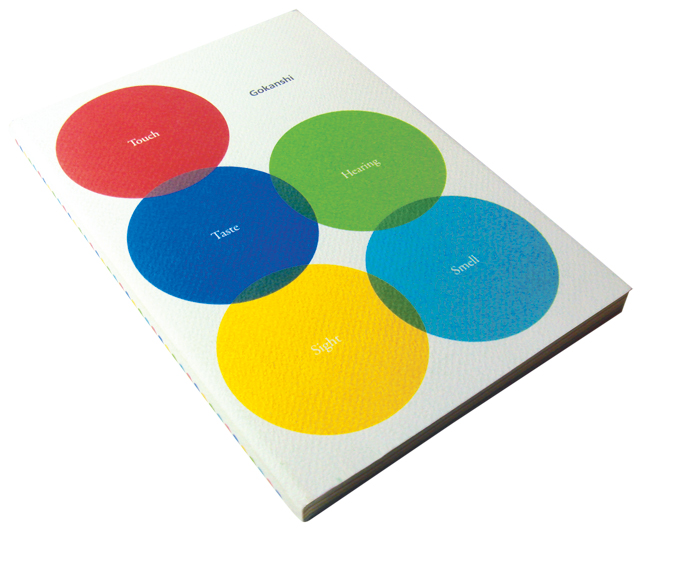This two notebooks were produced by recycle paper, and the paper¡¦s name (Gokanshi - Five Senses Paper from Japan) indicates that we can sense this paper¡¦s elasticity and thickness when we leaf over it. So I used five different colors to represent each sense, and repeated the numerous green dots to create the image of tree - the fundamental material of paper.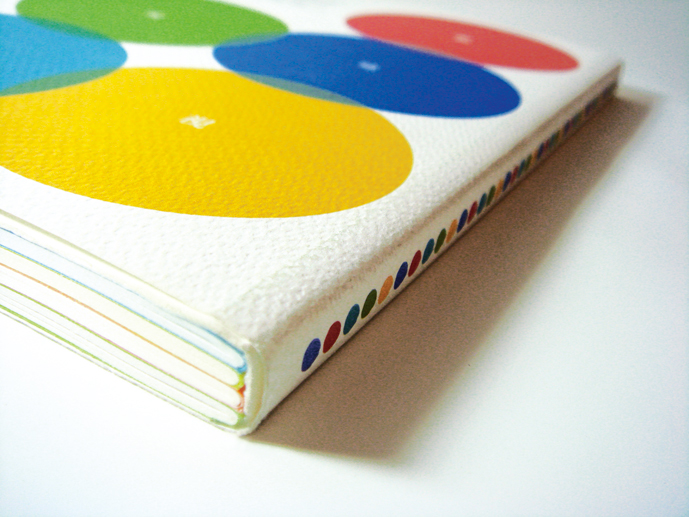This two notebooks were produced by recycle paper, and the paper¡¦s name (Gokanshi - Five Senses Paper from Japan) indicates that we can sense this paper¡¦s elasticity and thickness when we leaf over it. So I used five different colors to represent each sense, and repeated the numerous green dots to create the image of tree - the fundamental material of paper.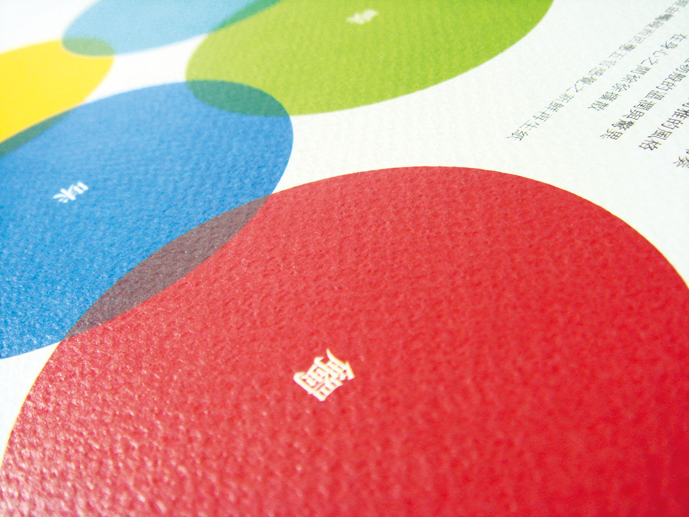This two notebooks were produced by recycle paper, and the paper¡¦s name (Gokanshi - Five Senses Paper from Japan) indicates that we can sense this paper¡¦s elasticity and thickness when we leaf over it. So I used five different colors to represent each sense, and repeated the numerous green dots to create the image of tree - the fundamental material of paper.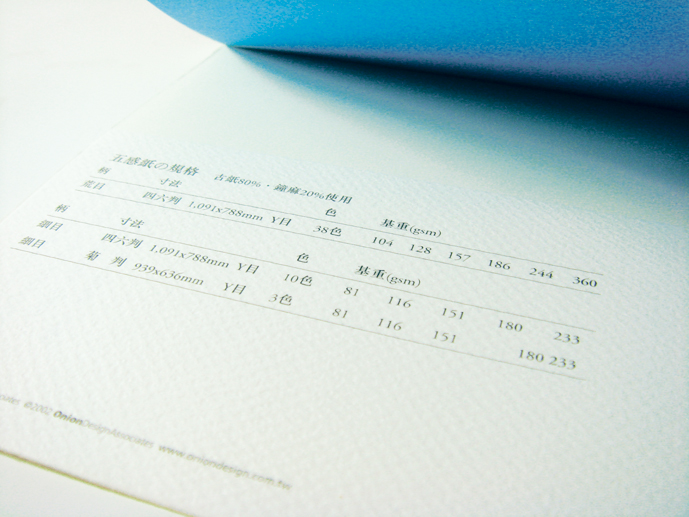This two notebooks were produced by recycle paper, and the paper¡¦s name (Gokanshi - Five Senses Paper from Japan) indicates that we can sense this paper¡¦s elasticity and thickness when we leaf over it. So I used five different colors to represent each sense, and repeated the numerous green dots to create the image of tree - the fundamental material of paper.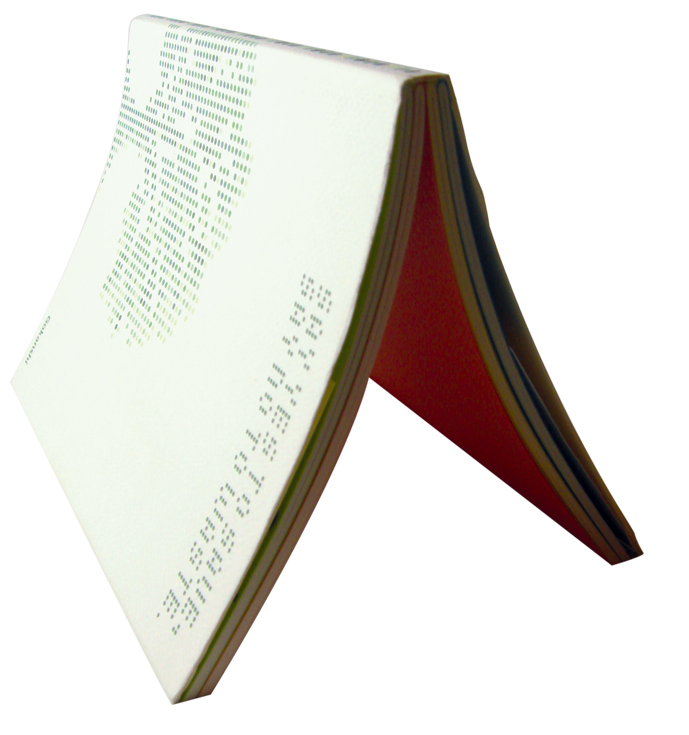This two notebooks were produced by recycle paper, and the paper¡¦s name (Gokanshi - Five Senses Paper from Japan) indicates that we can sense this paper¡¦s elasticity and thickness when we leaf over it. So I used five different colors to represent each sense, and repeated the numerous green dots to create the image of tree - the fundamental material of paper.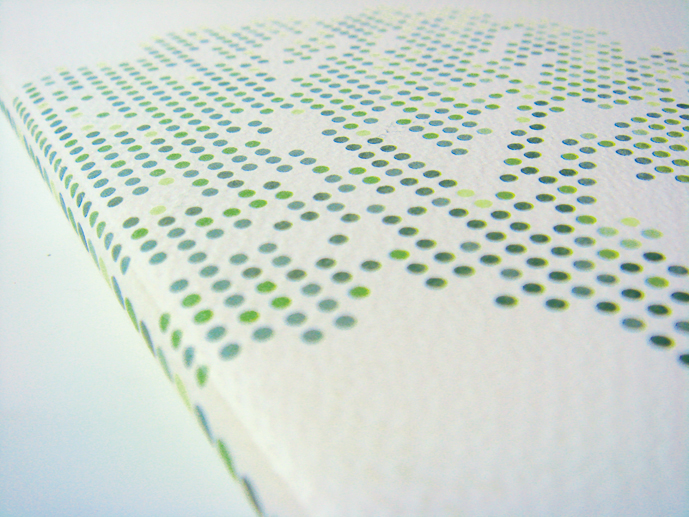This two notebooks were produced by recycle paper, and the paper¡¦s name (Gokanshi - Five Senses Paper from Japan) indicates that we can sense this paper¡¦s elasticity and thickness when we leaf over it. So I used five different colors to represent each sense, and repeated the numerous green dots to create the image of tree - the fundamental material of paper.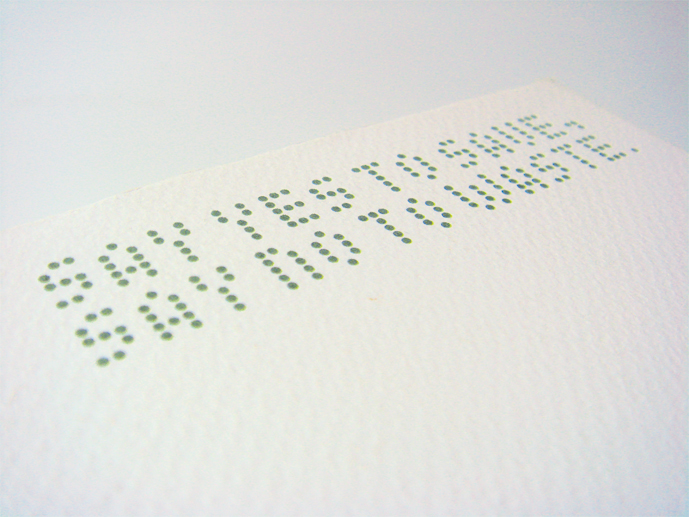This two notebooks were produced by recycle paper, and the paper¡¦s name (Gokanshi - Five Senses Paper from Japan) indicates that we can sense this paper¡¦s elasticity and thickness when we leaf over it. So I used five different colors to represent each sense, and repeated the numerous green dots to create the image of tree - the fundamental material of paper.
Auspic Paper Company - Promotional Notebook
Ralph Wu
Administrator at The Hole 台北, Taiwan